# Olivia Caramello's website

## Unifying theory

### Toposes as 'bridges'

#### General overview

At the beginning of my Ph.D. studies, I had the intuition that Grothendieck toposes could effectively serve as 'bridges' for connecting different mathematical theories with each other and allowing an effective transfer of knowledge between them. I therefore decided to undertake a systematic study of Grothendieck toposes with the aim of finding technical evidence to support this view, as well as of bringing into the context of Logic a whole set of powerful techniques of geometrical flavour, arising from a natural extension of Grothendieck's methodologies in Algebraic Geometry to the realm of first-order Mathematics.

In fact, the results obtained during my Ph.D. investigations, as well as in the following years, provided compelling technical evidence for the validity of this view, in the form of a number of non-trivial applications pertaining to different fields of Mathematics, such as Algebra, Geometry, Topology, Functional Analysis, Model Theory and Proof Theory (and of the solution of long-standing problems in Categorical Logic).

These results in turn stimulated further developments of the original idea, leading to a systematic set of techniques for studying mathematical theories by using toposes, which I summarized in the programmatic paper "The unification of Mathematics via Topos Theory".

As explained in that paper, as well as more briefly in this website, the principles introduced in this work are abstract and transversal to the various mathematical fields, and the application of them can lead to a great number of surprising and non-trivial results in different areas of Mathematics.

These methodologies are centered around the concept of Grothendieck topos. The theory of Grothendieck toposes is written in categorical language, but, unlike Category Theory, it is much more expressive, due to an additional degree of freedom implicit in the definition of topos. Indeed, a category can be thought of as a pair of sets related by some structure satisfying certain properties; any set can be regarded as a category, but most of the categories arising in Mathematics are not of this form. In fact, the concept of category has, with respect to the notion of set, an additional degree of freedom. Toposes are mathematical objects which are built from a pair, called a site, consisting of a category and a generalized covering, called Grothendieck topology, on it in a certain canonical way (the process which produces a topos from a given site can be described as a sort of 'completion'). Different coverings can be considered on a given category leading in general to inequivalent toposes; this gives the notion of topos one more degree of freedom with respect to that of category. The existence of these three 'degrees of freedom' implicit in the concept of a topos (two for the notion of category and one for that of Grothendieck topology) can be exploited to build 'mathematical universes' in which mathematical theories find their natural home and can be effectively compared with each other.

In fact, to any mathematical theory (of a general specified form) one can canonically associate a topos, called the classifying topos of the theory, which represents the natural framework in which the theory should be investigated, both in itself and in relationship to other theories. Two theories having the same classifying toposes (up to equivalence) are said to be Morita-equivalent.

The existence of theories which are Morita-equivalent to each other translates, at the level of sites, into the existence of different sites generating the same topos (up to equivalence); indeed, to any theory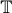one can canonically associate a site such that the topos built from it can be identified with its classifying topos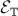.
The basic idea underlying the unification methodologies is that the classifying toposof a theorycan be effectively used as a 'bridge' to transfer information between the theoryand any other theory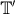which is Morita-equivalent to it:The transfer of knowledge between the two theories takes place as follows. For any given property or construction of toposes which is invariant under equivalence of toposes (one requires this invariance because the classifying topos is determined only up to equivalence), one tries to express it first in terms of one theory and then in terms of the other; provided that one obtains appropriate characterizations connecting properties of theories and properties of their classifying toposes (equivalently, characterizations connecting properties of sites and properties of toposes), this will lead to a logical relationship between properties of the two theories written in their respective languages.

A striking aspect of this technique is, besides its level of generality (indeed, it can be applied to mathematical theories belonging essentially to any mathematical field), the fact that it can be automatized in many cases. Indeed, using the methods of Topos Theory one can obtain characterizations of the above kind for several invariants, holding uniformly for any theory or at least for wide classes of theories (and for certain classes of invariants such characterizations can even be established in a purely mechanical way); in presence of a Morita-equivalence, these characterizations will thus be able to act as the arches of a bridge connecting the two theories, making it possible to transfer information between them.

#### The unifying methodologies

The fundamental intuition underlying the unifying methodologies is that a given mathematical property can manifest itself in several different forms in the context of mathematical theories which have a common semantics but a different linguistic presentation.

A (Grothendieck) topos can be thought of as a mathematical object which condenses in itself the semantics of a mathematical theory, representing the body of properties of the theory which do not depend on its linguistic presentation but only on its semantics. To any mathematical theory (of a general specified form) one can associate a topos, canonically built from any presentation of it, which represents its 'semantical core'. Such an object is called the classifying topos of the theory.

Two mathematical theories have the same classifying topos (up to equivalence) if and only if they have the same 'semantical core', that is if and only if they are indistinguishable from a semantic point of view; such theories are said to be Morita-equivalent. Conversely, every Grothendieck topos arises as the classifying topos of some theory.

Many important dualities and equivalences in Mathematics can be naturally interpreted in terms of equivalences between the classifying toposes of different theories; on the other hand, Topos Theory itself is a primary source of Morita-equivalences. It is fair to say that the notion of Morita-equivalence formalizes in many situations the feeling of 'looking at the same thing in different ways', and in fact it is ubiquitous in Mathematics.

Now, Grothendieck toposes can serve as 'bridges' for transferring information between Morita-equivalent theories in the following sense. The existence of different presentations for the 'semantical core' of a given mathematical theory translates, at the technical level, into the existence of different representations (technically speaking, sites) for the classifying topos of the theory. Topos-theoretic invariants, that is properties of toposes or constructions on them which are invariant under equivalence, can then be used to transfer properties between the two representations of the classifying topos and hence between the two theories. The transfer of properties arises from the process of expressing properties of toposes in terms of properties of their representations: these relationships between properties of sitesand properties of toposes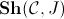are called site characterizations.

Given a Morita-equivalence and a topos-theoretic invariant I, site characterizations for I play in this context the role of the 'arches' of the bridge, the 'deck' of the bridge being given by the equivalence of toposes expressing the Morita-equivalence. The topos-theoretic invariant I thus acts in this context as a tool enabling to bring certain information from one side of it to the other of the equivalence; different invariants allow us to transfer different information.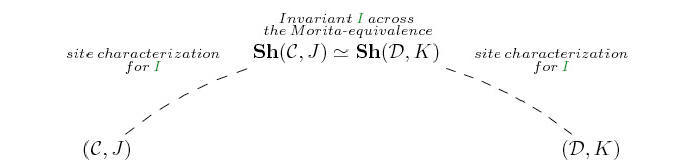This methodology is very effective from a technical viewpoint due to the fact that the relationship between a siteand the toposwhich it generates is technically very well-behaved (although highly non-trivial), to the extent that for many important classes of invariants there exist natural bijective site characterizations holding uniformly for any site, or at least for large classes of them. Also, there exist infinitely many topos-theoretic invariants (and one can always easily introduce new ones - since, by an obvious metatheorem, any property written in the language of Topos Theory is automatically invariant with respect to the notion of equivalence of toposes, namely categorical equivalence - and seek natural site characterizations for them).

We can thus think of a topos as an object which, together with all its different representations, embodies in itself a great amount of relationships existing between the different theories classified by it. Any topos-theoretic invariant thus behaves like a 'pair of glasses' which allows to discern certain information which is 'hidden' in a given Morita-equivalence.

These methods unify distinct mathematical theories with each other in the sense that properties (resp. constructions) arising in the context of theories which have a common 'semantical core' but a different 'linguistic presentation' come to be seen as different manifestations of a unique property (resp. construction) lying at the topos-theoretic level.

As a matter of fact, these methodologies define a novel way of doing Mathematics which is inherently 'upside-down' with respect to the more traditional approaches in which one starts with simple ingredients and proceeds to combine them to build more complicated structures. Indeed, these methodologies take as primitive ingredients rich and sophisticated mathematical entities, namely Morita-equivalences and topos-theoretic invariants, and allow one to extract from them a huge amount of information relevant for classical mathematics (it should however be noted that the fact that these 'primitive ingredients' are intrinsically complex does not imply that it is difficult to obtain such objects from the current mathematical practice, as it can be seen from my papers).

This intrinsically 'top-down' approach is actually liable to generate a great number of surprising insights into a variety of different mathematical contexts, a sort of uniform 'rain of results' into distinct subfields, results which could well be hard to establish, or even to merely imagine, by using the specialized methods of the different fields. As in the case of rain it is difficult, if not impossible, to predict where any single drop of it will fall, so in the case of the unifying methodologies it is hard to predict with certainty and exactness which results will be generated by the abstract machinery; on the other hand, as the power of the rain lies in the energy and matter which it globally generates, as well as in its uniform nature, so the power of the unifying methodologies lies in their 'global fruitfulness' and 'uniform' ramifications spreading transversally across the various subfields of Mathematics.

In fact, the creative power of these methodologies, as well as their uniform and canonical nature, makes them effective tools for assisting specialists of the various domain in the discovery of specific results, as well as a concrete guide for developing mathematics in a cohesive and conceptually inspired way.

Incidentally, it should be noted that these methodologies could also be generalized to the case of 'bridges' whose deck is given by some kind of relationship between toposes which is not necessarily an equivalence, in the presence of properties or constructions of toposes which are invariant with respect to such a relation.  Nonetheless, the advantage of focusing on Morita-equivalences is twofold; from a technical vewipoint, it is convenient because all natural topos-theoretic properties that one can formulate are automatically invariant with respect to categorical equivalence of toposes, while from a conceptual viewpoint it can be naturally interpreted as a unifying technique, since the properties of the different theories come to be interpreted as different manifestations of a unique property lying at the topos-theoretic level.

The unifying methodologies are also effective from a computational viewpoint. Indeed, the well-behaved representation theory of Grothendieck toposes allows to translate properties and results between different theories in a uniform and 'semi-automatic way'. In particular, one can generate a great number of non-trivial insights in different mathematical fields without really making any real creative effort. Of course, not all of the results generated in this way are necessarily 'interesting mathematical theorems'; many of them can be rather 'weird' according to usual mathematical standards, and in fact, in order to get interesting results, one has to use one's mathematical sensitivity to 'programme the machine' appropriately (that is, by choosing topos-theoretic invariants and Morita-equivalences in a sensible way); but these results can still be quite deep, due to the non-trivial nature of the representation theory of Grothendieck toposes.

Toposes can thus act as 'universal translators' across different mathematical theories in a very effective and fruitful way. From a technical point of view, the main reason for this effectiveness is two-fold: on one hand, topos-theoretic invariants usually manifest themselves in significantly different ways in the context of different sites (think for example of the amalgamation property on a category or the property of a topological space to be extremally disconnected, which represent different manifestations of a given topos-theoretic invariants, namely the property of a topos to satisfy De Morgan's law, in the context of different sites - see here for more details), while on the other hand the site characterizations for them which formally express such manifestations are essentially canonical and can be generally established in a systematic, and in many cases even mechanical, way, due to the very well-behaved nature of the representation theory of Grothendieck toposes in terms of sites.

Concerning the level of 'mathematical depth' of the translations established through the bridge technique, we remark that it is clearly impossible to accurately predict it a priori. Anyway, an upper bound for the complexity of such results is given by the sum of the mathematical depth of the three parts of the bridge, namely its 'deck' (given by the Morita-equivalence) and its two 'arches' (given by the site characterizations), which nonetheless can be very considerable (due to the depth of topos-theoretic methods).

Actually, while in most cases the topos-theoretic translations do not admit easy or natural elementary proofs, it may happen that, after discovering a certain 'translation' through the bridge method, one could find a 'concrete' proof of it which does not involve toposes; but even in these cases one often realizes that, in spite of its greater technical complexity, it is the topos-theoretic proof that best illuminates the 'real reason' why the result is true, and also that ultimately it would have been rather hard, if not impossible, to arrive at the result or even imagine it without the 'guide' of toposes. Indeed, the topos-theoretic proofs arising from applications of the bridge method are natural both from a technical viewpoint (because of their canonicity) and from a conceptual one (because of their unifying nature, which reveals itself in the fact that the method relates to each other properties (or constructions) which represent different concrete manifestations of a unique abstract property (or construction) lying at a higher level.

In a sense, the 'upside-down' way in which the results are generated through the bridge method is so different from the traditional techniques that it defines by itself a new way of doing Mathematics, intrinsically intra-disciplinary in character, which owes its strength to the identification of 'centres of mathematical symmetry' from which mathematical reality can be naturally observed. In fact, the naturality of this point of view is witnessed by its technical fruitfulness and creative power, which reflects itself in the possibility of a 'canonical' and computationally effective generation of concrete applications in distinct mathematical domains.